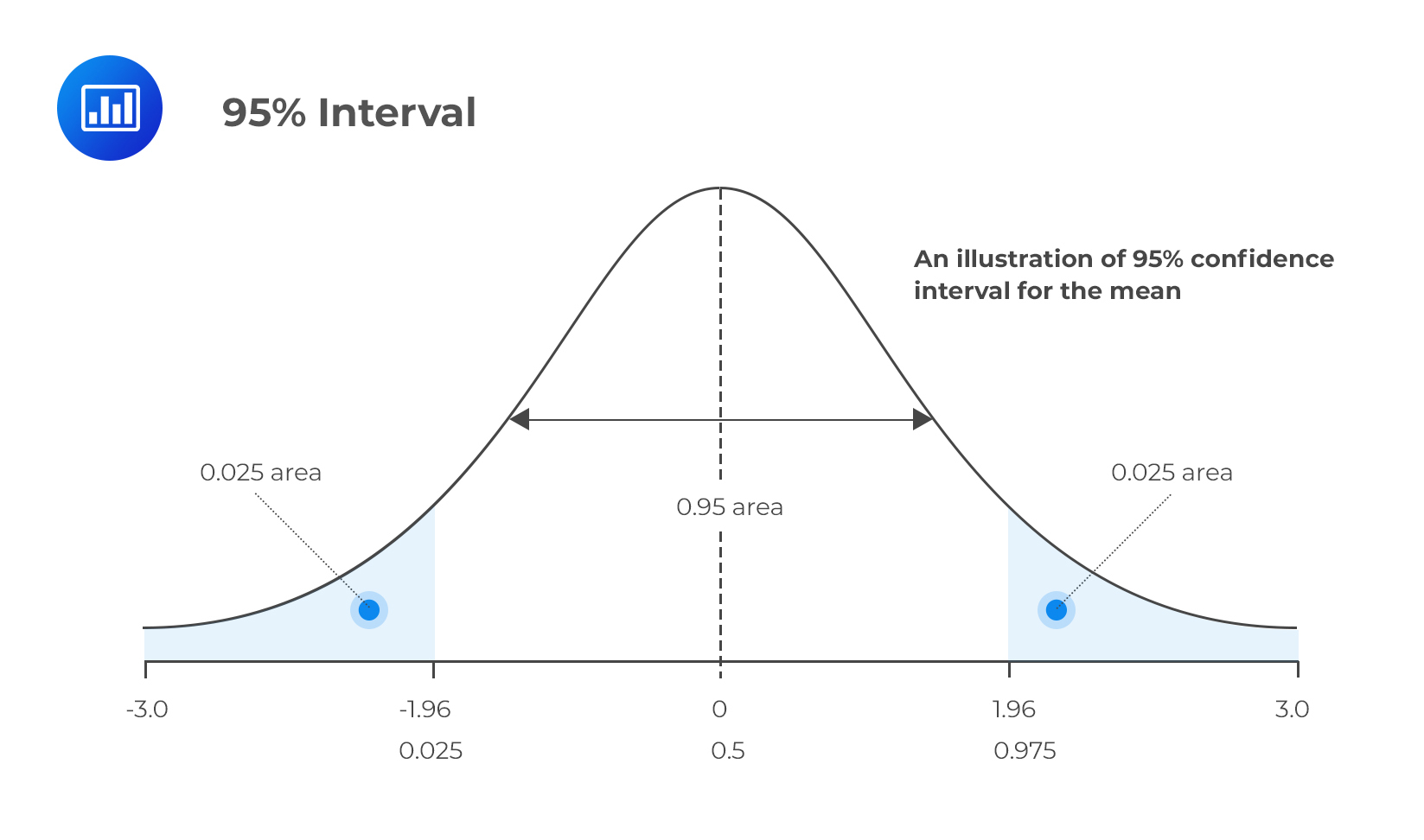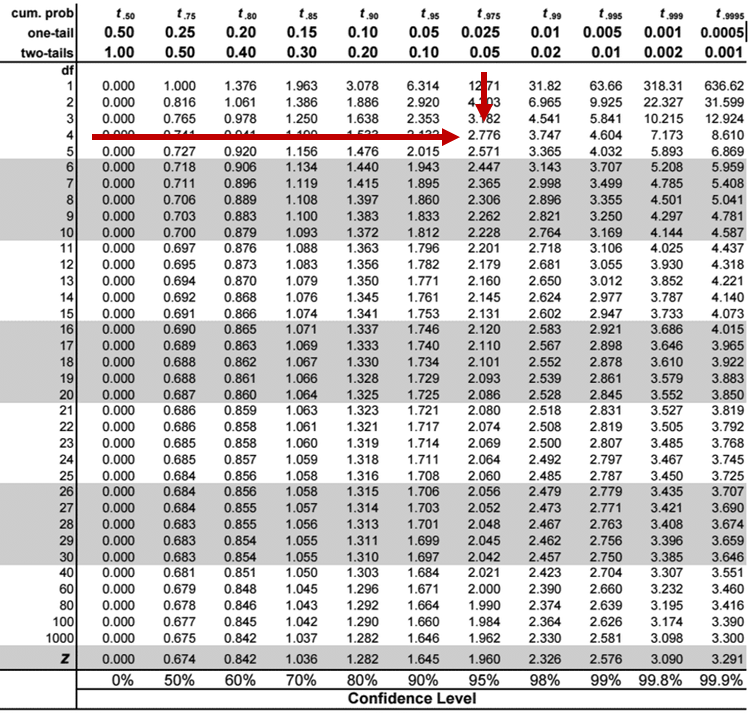Limited Time Offer: Save 10% on all 2021 and 2022 Premium Study Packages with promo code: BLOG10    Select your Premium Package »Confidence Intervals

Confidence interval (CI) refers to a range of values within which statisticians believe the actual value of a certain population parameter lies. It is different from from a point estimate which is a single, specific numerical value.

Breaking Down Confidence Interval

When constructing confidence intervals, we must specify the probability that the interval contains the true value of the parameter of interest. This probability is represented by (1 – α), where α is the level of significance. In statistical terminology, (1- α) is called the degree of confidence or certainty.

We define a 100 (1 – α)% confidence interval for a given parameter, say θ, by specifying two random variables, θ’1(X) and θ’2(X), such that P{θ’1(X) < θ < θ’2(X)} = 1 – α.

It happens that α = 0.05 is the most common case in examinations and practice. This leads to a 95% confidence interval.

Consequently, P{θ’1(X) < θ < θ’2(X)} = 0.95 specifies {θ’1(X), θ’2(X)} as a 95% confidence interval for θ. The main task for a candidate lies in being able to construct and interpret a confidence interval. Therefore, the CI for θ above could be interpreted to mean that if we were to construct similar intervals using samples of equal sizes from the same population, 95% of the intervals would contain the true parameter value and just 5% would not contain it, hence, the phrase “confidence” interval.

Constructing Confidence Intervals

To construct a confidence interval, one must come up with an appropriate value that will be subtracted and added to a point estimate. A confidence interval appears as follows:

$$\text{CI} =\text{Point estimate} \pm \text{Reliability factor} × \text{Standard error}$$

Where:

The point estimate refers to a calculated value of the sample statistic such as the mean, X.

The reliability factor is a value that depends on the sampling distribution involved and (1 – α), the probability that the point estimate is contained in the confidence interval.

The Standard error is the standard error of the point estimate.

Different Scenarios

1. Normal Distribution With a Known Variance

We can calculate the confidence interval for the mean as:

$$x \pm z_{\alpha/2} × \frac {\sigma}{\sqrt n}$$

Here, the reliability factor is zα/2. The z-score leaves a probability of α/2 on the upper tail (right-hand tail) of the standard normal distribution.

The table below represents the standard normal distributions commonly used by analysts.

$$\begin{array}{c|c|c} \text{Degree of confidence} & \text{Level of significance(one-tailed)} & {z_{\alpha/2}} \\ \hline {90\%} & {10\%} & {1.645} \\ \hline {95\%} & {5\%} & {1.960} \\ \hline {99\%} & {1\%} & {2.575} \\ \end{array}$$2. Normal Distribution With Unknown Variance

When the variance is unknown, we construct the confidence interval for the mean by replacing the z-score in the first scenario with the t-score. Similarly, we replace the unknown σ with S, the standard deviation of the sample mean. Therefore,

$$CI = x \pm t_{\alpha/2} × \frac {S}{\sqrt n}$$

tα/2 is the t-score that leaves a probability of α/2 on the upper tail of the t-distribution. The number of degrees of freedom is determined by the sample size such that the degrees of freedom (df) = n – 1.

3. Any Distribution When Variance is Unknown, and the Sample Size is Large Enough

Thanks to the Central Limit Theorem, we can approximate just about any non-normal distribution the same way we do a normal one, provided the sample size is large (n ≥ 30). Furthermore, we can use the relevant z-score when constructing a confidence interval for the population mean.

However, some analysts may advocate for the use of a t-distribution in scenarios where the distribution is non-normal, and the population variance is unknown, even if n ≥ 30. Regardless of such arguments, the use of the z statistic would still be justified under such circumstances, provided the central limit theorem is applied correctly.

Example: Confidence Interval

A teacher draws a sample of five 12-year-old children from a school’s population and records their heights in centimeters as follows:

$$\{124, 124, 128, 130, 127\}$$

Assume that the heights have a normal distribution where both μ and σ are unknown. Calculate a two-tailed 95% confidence interval for the mean height of 12-year-olds.

Solution

Since the variance is unknown and the sample size is less than 30, we should use the t-score instead of the z-score, even if the distribution is normal. As such, we will calculate the confidence interval for the mean as follows:

$$CI = x \pm t_{\alpha/2} × \frac {S}{\sqrt n}$$

From the data, X = 126.6 and S2 = 6.8

You can read off the t-score value from the t-distribution table where you will find that,

$$t_{4, 0.025} = 2.776$$

Please, refer to the t-table below to find the critical t-value.Therefore,

\begin{align*} CI & = 126.6 \pm 2.776 \times \frac {\sqrt 6.8}{\sqrt 5} \\ & = 126.6 \pm 3.2373 \\ \end{align*}

Therefore, our confidence interval for μ is (123.36, 129.84).

Question

Use the data from the example above to calculate a two-tailed 99% confidence interval for the population mean.

1. (125.3, 127.91)
2. (117.9, 135.3)
3. (116.6, 136.6)

Solution

$$CI = x \pm t_{\alpha/2} × \frac {S}{\sqrt n}$$

$$t_{4, 0.005 }= 4.604$$

The other inputs remain the same as in the example above.

Therefore,

\begin{align*} CI & = 126.6 \pm 4.604× \frac {\sqrt 6.8}{\sqrt 5} \\ & = 126.6 \pm 5.4391 \\ \end{align*}

The confidence interval for the mean is (121.16, 132.01).

As you might have observed, the interval widens as the level of confidence increases.

Featured Study with UsCFA® Exam and FRM® Exam Prep Platform offered by AnalystPrep

Study Platform

Learn with Us

Subscribe to our newsletter and keep up with the latest and greatest tips for success
Online TutoringOur videos feature professional educators presenting in-depth explanations of all topics introduced in the curriculum.

Video LessonsSergio Torrico
2021-07-23
Excelente para el FRM 2 Escribo esta revisión en español para los hispanohablantes, soy de Bolivia, y utilicé AnalystPrep para dudas y consultas sobre mi preparación para el FRM nivel 2 (lo tomé una sola vez y aprobé muy bien), siempre tuve un soporte claro, directo y rápido, el material sale rápido cuando hay cambios en el temario de GARP, y los ejercicios y exámenes son muy útiles para practicar.diana
2021-07-17
So helpful. I have been using the videos to prepare for the CFA Level II exam. The videos signpost the reading contents, explain the concepts and provide additional context for specific concepts. The fun light-hearted analogies are also a welcome break to some very dry content. I usually watch the videos before going into more in-depth reading and they are a good way to avoid being overwhelmed by the sheer volume of content when you look at the readings.Kriti Dhawan
2021-07-16
A great curriculum provider. James sir explains the concept so well that rather than memorising it, you tend to intuitively understand and absorb them. Thank you ! Grateful I saw this at the right time for my CFA prep.nikhil kumar
2021-06-28
Very well explained and gives a great insight about topics in a very short time. Glad to have found Professor Forjan's lectures.Marwan
2021-06-22
Great support throughout the course by the team, did not feel neglectedBenjamin anonymous
2021-05-10
I loved using AnalystPrep for FRM. QBank is huge, videos are great. Would recommend to a friendDaniel Glyn
2021-03-24
I have finished my FRM1 thanks to AnalystPrep. And now using AnalystPrep for my FRM2 preparation. Professor Forjan is brilliant. He gives such good explanations and analogies. And more than anything makes learning fun. A big thank you to Analystprep and Professor Forjan. 5 stars all the way!michael walshe
2021-03-18
Professor James' videos are excellent for understanding the underlying theories behind financial engineering / financial analysis. The AnalystPrep videos were better than any of the others that I searched through on YouTube for providing a clear explanation of some concepts, such as Portfolio theory, CAPM, and Arbitrage Pricing theory. Watching these cleared up many of the unclarities I had in my head. Highly recommended.